## ML Aggarwal Class 6 Solutions for ICSE Maths Chapter 1 Knowing Our Numbers Ex 1.3

Question 1.
In a particular year, a company manufactured 8570435 bicycles and next year it manufactured 8756430 bicycles. In which year more bicycles were manufactured and by how many?
Solution:
Production of bicycles in a particular year
= 85,70,435
Production of bicycles in a next year
= 87,56,430
Both the numbers 85,70,435 and 87,56,430 are 7 digit numbers and 87 > 85
∴ 87,56,430 > 85,70,435
∴ Their Difference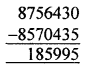Hence, the production of bicycles in a next year is more and by 1,85,995 cycles of 87,56,430.

Question 2.
What number must be subtracted from 1,02,59,756 to get 77,63,835?
Solution:
First number = 1,02,59,756
Resultant number = 77,63,835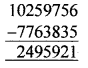The number to be subtracted from first number to obtain the resultant number is 24,95,921.

Question 3.
The sale receipt of a company during a year was ₹ 30587850. Next year it increased by ₹ 6375490. What was the total sale receipt of the company during these two years?
Solution:
Sale in the first year = ₹ 30587850
Sale increased in the next year by = ₹ 6375490
∴ Sale in the second year
= ₹ 30587850 + ₹ 6375490
= ₹ 36963340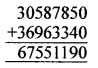Total sale for the two years = ₹ 67551190

Question 4.
A machine manufactures 23875 screws per day. How many screws did it produce iff the year 2012? Assume that the machine worked on all the days of the year.
Solution:
Production of screws per day = 23875
2012 is a leap year, so there are 366 days
Total production of screws per year
= 23875 × 366 = 8738250Question 5.
A merchant had ₹ 78,592 with him. He placed an order for puchasing 54 bicycles at ₹ 970 each. How much money will remain with him after the purchase?
Solution:
Total money available with merchant = ₹ 78,592
Total money paid for purchasing 54 bicycles at ₹ 970 = ₹ 52,380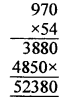Money left with merchant
= ₹ 78,592 – ₹ 52,380 = ₹ 26,212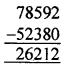Question 6.
Amitabh is 1 m 82 cm tall and his wife is 35 cm shorter than him. What is his wife’s height?
Solution:
Height of Amitabh = 1 m 82 cm
= 1 × 100 + 82 cm = 182 cm
Amitabh wife s 35 cm shorter than Amitabh
∴ Amitabh wife height = 182 – 35 = 147 cm

Question 7.
The mass of each gas cylinder is 21 kg 270g. What is total mass of 28 such cylinders?
Solution:
Mass of one gas cylinder = 21 kg 270 g
= 21 × 1000 + 270 g
= 21270g
Total mass of 28 gas cylinder = 21270 × 28
= 595560 g
= 59 kg 556 g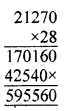Question 8.
In order to make a shirt, 2 m 25 cm cloth is needed. What length of cloth is required to make 18 such shirts?
Solution:
The length of cloth required to stitch one shirt = 2 m 25 cm
= 2 × 100 + 25 cm = 225 cm
The length of cloth required to stitch 18 shirts
= 225 × 18 = 4050 cm
= 40 m 50 cm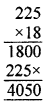Question 9.
The total mass of 12 packets of sweets, each of the same size, is 15 kg 600 g. What is the mass of each such packet?
Solution:
Total mass of 12 packets of sweets
= 15 kg 600 g
= 15 × 1000 + 600 g
= 15600 g
Total mass of one packet of sweets
= 15600 – 12 = 1300 g
= 1 kg 300 gQuestion 10.
A vessel has 4 litres 500 millilitres of orange juice. In how many glasses, each of 25 mL capacity, can it be filled?
Solution:
Total capacity of vessel containing orange juice = 4 litres 500 mL
= 4 × 1000 + 500 mL
= 4500 mL
Size of glass = 25 mL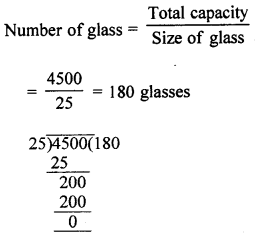Question 11.
To stitch a trouser, 1 m 30 cm cloth is needed. Out of 25 m cloth, how many trousers can be stitched and how much cloth will remain?
Solution:
Total length of cloth available = 25 m
= 25 × 100 = 2500 cm
The length of cloth required to stitch a trouser
= 1m 30 cm
= 100 + 30 cm = 130 cm
Dividing 2500 by 130, we get quotient = 19 and remainder = 30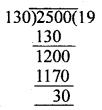∴ The number of trousers that can be stitched = 19
and the length of the remaining cloth = 30cm